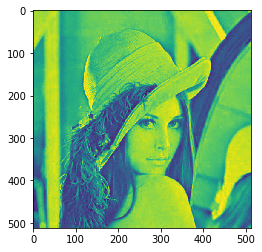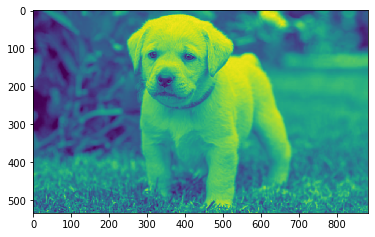Related Articles

# Mahotas – Roundness of Image

• Last Updated : 31 May, 2021

In this article we will see how we can get the image roundness feature in mahotas. Roundness is dominated by the shape’s gross features rather than the definition of its edges and corners, or the surface roughness of a manufactured object. A smooth ellipse can have low roundness, if its eccentricity is large
For this tutorial we will use ‘lena’ image, below is the command to load the lena image

`mahotas.demos.load('lena')`

Below is the lena imageIn order to do this we will use mahotas.features.roundness method
Syntax : mahotas.features.roundness(img)
Argument : It takes image object as argument
Return : It returns float value

Note : Input image should be filtered or should be loaded as grey
In order to filter the image we will take the image object which is numpy.ndarray and filter it with the help of indexing, below is the command to do this

`image = image[:, :, 0]`

Below is the implementation

## Python3

 `# importing required libraries``import` `mahotas``import` `mahotas.demos``from` `pylab ``import` `gray, imshow, show``import` `numpy as np``import` `matplotlib.pyplot as plt``  ` `# loading image``img ``=` `mahotas.demos.load(``'lena'``)``  ` `# filtering image``img ``=` `img.``max``(``2``)` `print``(``"Image"``)``  ` `# showing image``imshow(img)``show()` `# computing roundness in image``value ``=` `mahotas.features.roundness(img)`` `  `# printing value``print``(``"Roundness : "` `+` `str``(value))`

Output :

`Image``Roundness : 0.0`

Another example

## Python3

 `# importing required libraries``import` `mahotas``import` `numpy as np``from` `pylab ``import` `gray, imshow, show``import` `os``import` `matplotlib.pyplot as plt`` ` `# loading image``img ``=` `mahotas.imread(``'dog_image.png'``)`  `# filtering image``img ``=` `img[:, :, ``0``]``  ` `print``(``"Image"``)``  ` `# showing image``imshow(img)``show()` `# computing roundness in image``value ``=` `mahotas.features.roundness(img)`` `  `# printing value``print``(``"Roundness : "` `+` `str``(value))`

Output :

`Image``Roundness : 0.04297201733896709`

Attention geek! Strengthen your foundations with the Python Programming Foundation Course and learn the basics.

To begin with, your interview preparations Enhance your Data Structures concepts with the Python DS Course. And to begin with your Machine Learning Journey, join the Machine Learning – Basic Level Course

My Personal Notes arrow_drop_up